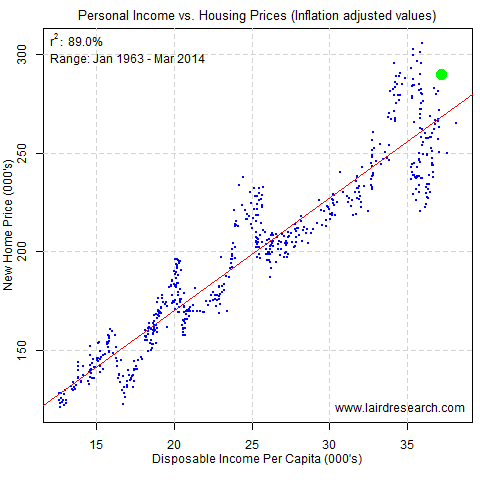# Are Housing Prices High?

Many US recoveries in the past have been driven by housing. Conversely, a major factor in the meltdown in 2008 was also driven by housing. It’s reasonable to ask: how can we identify housing bubbles?

Bubbles are tied to discussions about whether the current price levels are sustainable. There are a lot of ways to skin that particular cat, but one item I like to keep track of is the relation between housing prices and disposable income. There’s a clear linear relationship between the two and a very aggressive “reversion to mean” behaviour. Whether this is more about prices collapsing or incomes rising is up for debate and the trigger to make that happen is something I haven’t figured out, but its a great relationship to watch.The latest data point is the green dot. Note how far above the trendline it is sitting.

Let’s look at the data behind the chart. The best free source I can think of is FRED – it’s timely, comprehensive and easy to download with R. Here’s the specific series that I’m looking at:

``` # Load required data
library(quantmod)
getSymbols(c("MSPNHSUS", "CUUR0000SA0L2", "A229RX0"), src="FRED")
```

This shows new home pricing (MSPNHSUS) and disposable income per capita (A229RX0). Finally, since we are looking for such long periods of time, its worthwhile to take inflation into account, however I wanted to look at inflation excluding house pricing (CUUR0000SA0L2). Next step is to marshal the data into a nice format:

```# Calculate raw data
real.home.sales <- MSPNHSUS / CUUR0000SA0L2 * as.numeric(last(CUUR0000SA0L2))
real.ave.income <- A229RX0
house.data<-cbind(real.home.sales/1000, real.ave.income/1000)
names(house.data)<-c("real.home.sales","real.ave.income")
house.data <- as.data.frame(house.data[complete.cases(house.data),])

# simple linear model of home sales to average income
home.income.lm <- lm(real.home.sales ~ real.ave.income, data=house.data)
```

Now that we have the data in some nice data frames, here's the code to built the plot of the results above.

```# create the plot
par(mar=c(4,3,2,0.5), cex=1)
plot(x=house.data\$real.ave.income,
y=house.data\$real.home.sales,
ylab="", xlab="",main="", type="n")
grid(lty=2, col="lightgrey")
points(x=house.data\$real.ave.income,
y=house.data\$real.home.sales,
pch=20, col="blue", cex=0.5)
title(main="Personal Income vs. Housing Prices (Inflation adjusted values)",
cex.main=1.1, font.main=1)
mtext(side=2, "New Home Price (000's)", line=2)
mtext(side=1, "Disposable Income Per Capita (000's)", line=2)

# plot the latest point
last.point=c(last(house.data\$real.ave.income), last(house.data\$real.home.sales))
points(x=last.point, y=last.point, pch=20, col="green", cex=3)

# plot the best fit line
abline(home.income.lm, col="red")

text(x=par("usr"), y=par("usr")-strheight("R")*1.1,
pos=4, offset=0.5,